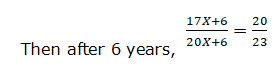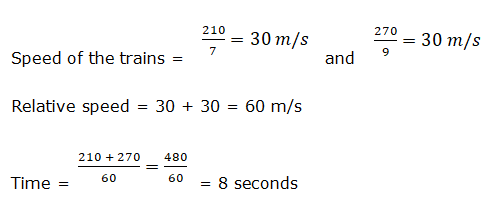# RRB Clerk Prelims Quantitative Aptitude Questions 2021 (Day-11)

Dear Aspirants, Our IBPS Guide team is providing new series of Quantitative Aptitude Questions for RRB Clerk Prelims 2021 so the aspirants can practice it on a daily basis. These questions are framed by our skilled experts after understanding your needs thoroughly. Aspirants can practice these new series questions daily to familiarize with the exact exam pattern and make your preparation effective.

Start Quiz

Ages

1) The ages of Ramesh and Ram are in the ratio of 17:20. After 6 years, the ratio of the ages of Ramesh to Ram will be 20:23. What was the age of Ramesh 6 years ago?

A.28 years

B.30 years

C.25 years

D.31 years

E.None of these

Trains

2) Two trains of length 210 m and 270 m respectively moving at some speed to pass a man who is standing on a platform in 7 seconds and 9 seconds respectively. In what time will they cross each other, if they are moving in opposite directions?

A.7 seconds

B.10 seconds

C.11 seconds

D.8 seconds

E.None of these

Percentage

3) The sum of the monthly salary of Anu and Sunil is Rs.7000 more than the monthly salary of Bindhu. If the ratio of the monthly salary of Anu and Sunil is 2:5 and the monthly salary of Bindhu is Rs.3500. The monthly salary of Anu is approximately what percent of the monthly salary of Bindhu?

A.85.7%

B.88.6%

C.92.5%

D.78.4%

E.None of these

Mixture and allegation

4) Vessel A contains 28 liters of milk and x liters of water. If 24 liters of mixture is taken out which is contains 8 liters of water, then find the value of x?

A.12 liters

B.14 liters

C.16 liters

D.18 liters

E.20 liters

Probability

5) A box contains x red ball, 5 green ball and 4 blue ball. If one ball is drawn at random from the box and the probability of that ball is red is 2/5, then what is the probability of 2 blue balls is drawn at random from the box?

A.2/35

B.1/35

C.3/35

D.4/35

E.1/7

Caselet

Directions (06-10): Study the following information carefully and answer the questions given below.

A company has four departments HR, IT, Sales and Finance. The number of male employees in HR department is double the number of female employees in IT department. The number of female employees in Finance department is 50% of the number of female employees in HR department. Total number of employees in Finance department is 360. The number of male employees in Sales department is 60 more than the number of female employees in Finance. The number of male employees in IT department is equal to the total number of employees in finance department. Total number of employees in HR is 80 more than the total number of employees in IT. The number of female employees in Sales is 50% of the number of male employees in IT department. The number of male to female employees in Sales department is 11:9.

6) What is the total number of employees in the company?

A.1800

B.1600

C.2000

D.1400

E.None of these

7) The number of female employees in IT is what percent of the total number of employees in Sales?

A.20%

B.25%

C.30%

D.35%

E.None of these

8) What is the difference between the total number of male employees and female employees in all the departments together?

A.210

B.220

C.230

D.240

E.None of these

9) The number of male employees in Finance
department is approximately what percent of the total number of employees in HR?

A.36%

B.39%

C.42%

D.45%

E.48%

10) Ratio of the number of undergraduate to postgraduate employees in Sales department is 3:2. Out of 45% of male employees in sales departments is postgraduate. Find the number of female postgraduate employees in Sales department.

A.59

B.61

C.63

D.65

E.67

Let Ramesh’s age be 17X and Ram’s age be 20X.391X + 138 = 400X + 120

9X = 18

X = 2

Thus, Ramesh’s present age = 17 x 2= 34 years

6 years ago Ramesh’s age = 34 – 6 = 28 yearsBindhu = Rs.3500

Anu = 2x

Sunil = 5x

2x + 5x – 3500 = 7000

7x = 10500

x = 1500

Anu = 2 * 1500 = 3000

Required percentage = 3000/3500 * 100 = 85.7%

Quantity of mixture = 24 liters

Water in mixture = 8 liters

Milk in mixture = 24 – 8 = 16 liters

Ratio of milk and water = 16:8 = 2:1

x = 1/2 * 28 = 14 liters

2/5 = xC1/(9 + x)C1

5x = 18 + 2x

x = 6

Required probability = 4C2/15C2

= 4 * 3/15 * 14

= 2/35

Directions (6-10) :

Number of male employees in IT department = 360

Number of female employees in Sales department = 360 * 50/100 = 180

Number of male employees in Sales department = 11/9 * 180 = 220

Number of female employees in Finance department = 220 – 60 = 160

Number of male employees in Finance department = 360 – 160 = 200

Number of female employees in HR department = 100/50 * 160 = 320

Number of female employees in IT department = x

Number of male employees in HR department = 2x

2x + 320 – (x + 360) = 80

x = 120

Number of female employees in IT department = 120

Number of male employees in HR department = 2 * 120 = 240

Total number of employees in HR department = 240 + 320 = 560

Number of employees in IT department = 360 + 120 = 480

Number of employees in Sales department = 220 + 180 = 400

Total number of employees = 560 + 480 + 400 + 360 = 1800

Required percentage = 120/400 * 100 = 30%

Total male employees = 240 + 360 + 220 + 200 = 1020

Total female employees = 320 + 120 + 180 + 160 = 780

Difference = 1020 – 780 = 240

Required percentage = 200/560 * 100 = 36%